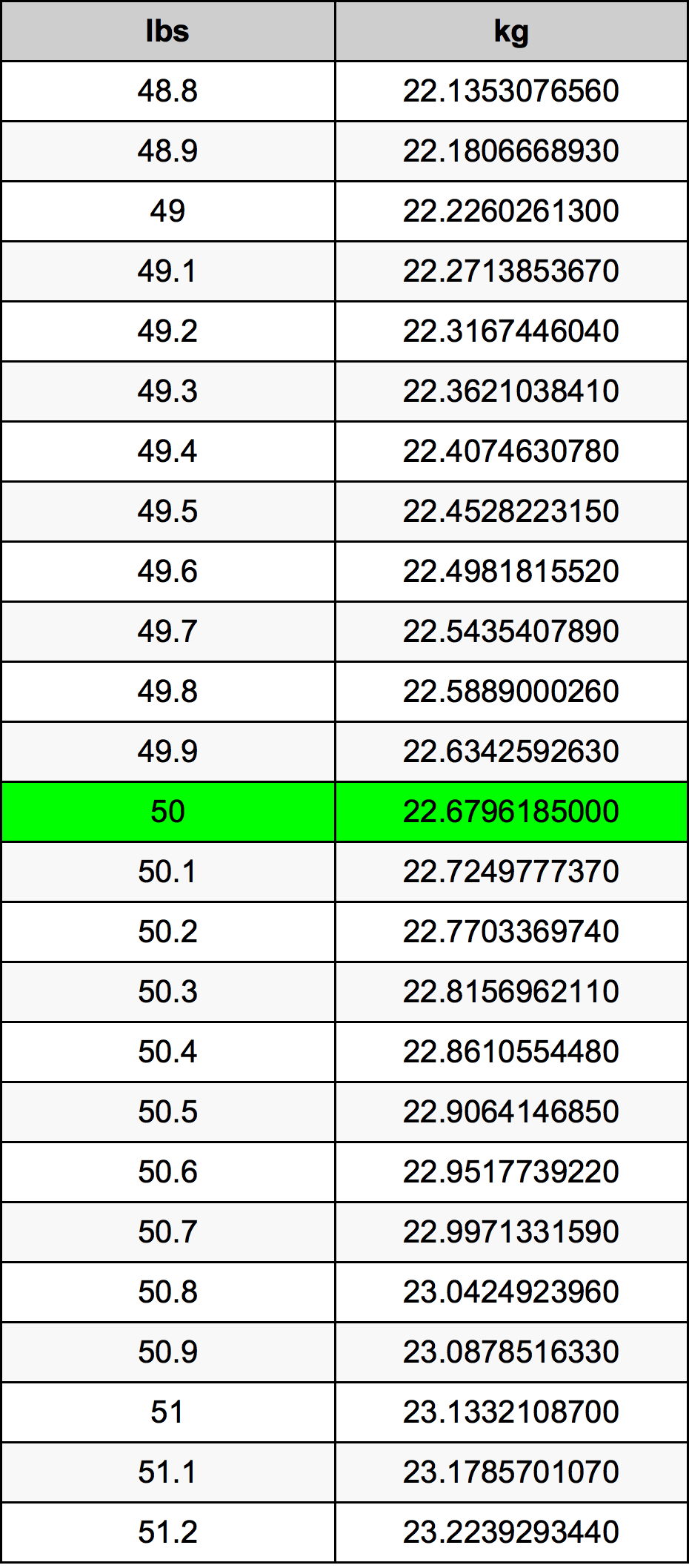Pounds To Kg

# 50 lbs to kg50 Pounds to Kilograms

lbs
=
kg

## How to convert 50 pounds to kilograms?

 50 lbs * 0.45359237 kg = 22.6796185 kg 1 lbs
A common question is How many pound in 50 kilogram? And the answer is 110.231131092 lbs in 50 kg. Likewise the question how many kilogram in 50 pound has the answer of 22.6796185 kg in 50 lbs.

## How much are 50 pounds in kilograms?

50 pounds equal 22.6796185 kilograms (50lbs = 22.6796185kg). Converting 50 lb to kg is easy. Simply use our calculator above, or apply the formula to change the length 50 lbs to kg.

## Convert 50 lbs to common mass

UnitMass
Microgram22679618500.0 µg
Milligram22679618.5 mg
Gram22679.6185 g
Ounce800.0 oz
Pound50.0 lbs
Kilogram22.6796185 kg
Stone3.5714285714 st
US ton0.025 ton
Tonne0.0226796185 t
Imperial ton0.0223214286 Long tons

## What is 50 pounds in kg?

To convert 50 lbs to kg multiply the mass in pounds by 0.45359237. The 50 lbs in kg formula is [kg] = 50 * 0.45359237. Thus, for 50 pounds in kilogram we get 22.6796185 kg.

## 50 Pound Conversion Table## Alternative spelling

50 Pounds to Kilogram, 50 Pounds in Kilogram, 50 Pound to Kilogram, 50 Pound in Kilogram, 50 Pounds to kg, 50 Pounds in kg, 50 Pound to Kilograms, 50 Pound in Kilograms, 50 lbs to Kilograms, 50 lbs in Kilograms, 50 lb to Kilograms, 50 lb in Kilograms, 50 lbs to Kilogram, 50 lbs in Kilogram, 50 Pound to kg, 50 Pound in kg, 50 lb to kg, 50 lb in kg